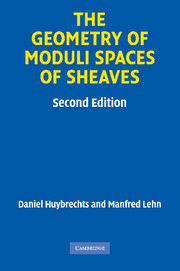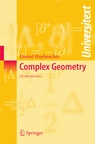### Books

1. Lectures on K3 surfaces. Cambridge University Press. 2016. 496 pages. Old homepage with pdf of early version and an erratum is here
2. Fourier-Mukai transforms in Algebraic Geometry. Oxford Mathematical Monographs. 2006. 307 pages.
3. Complex geometry - an introduction. Springer (2004). Universitext. 309 pages.
4. (with D. Joyce, M. Gross) Calabi-Yau manifolds and related geometries. Springer (2002), 244 pages.
5. (with M. Lehn) The geometry of moduli spaces of shaves. Aspects of Mathematics E 31, Vieweg (1997), 260 pages.

A second edition has appeared in the Cambridge Mathematical Library of Cambridge Unversity Press (2010).

6.